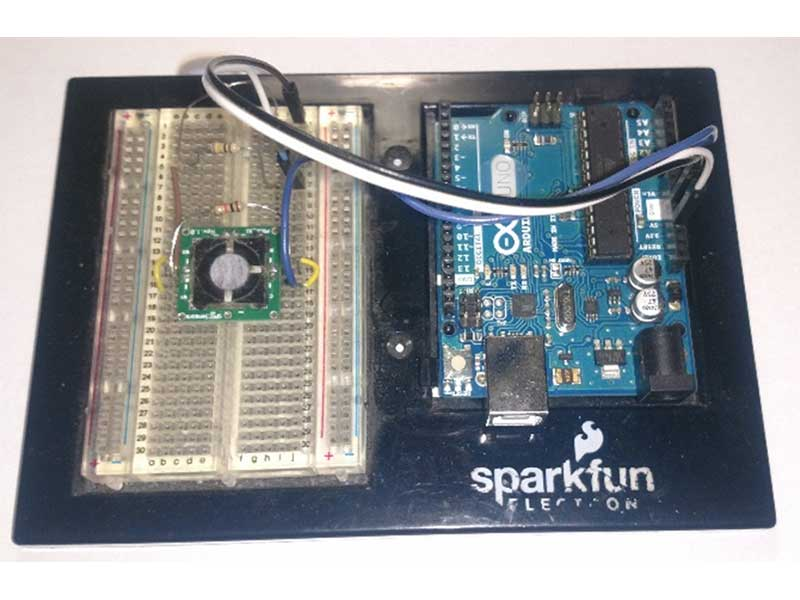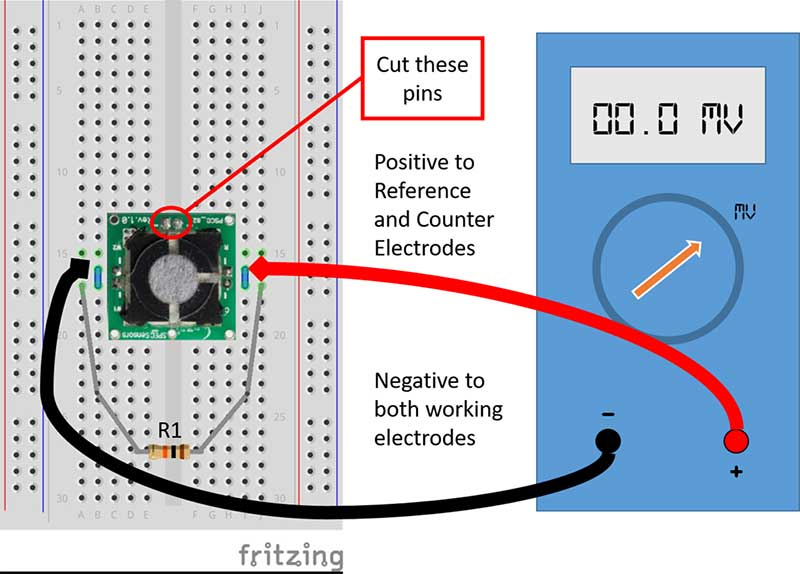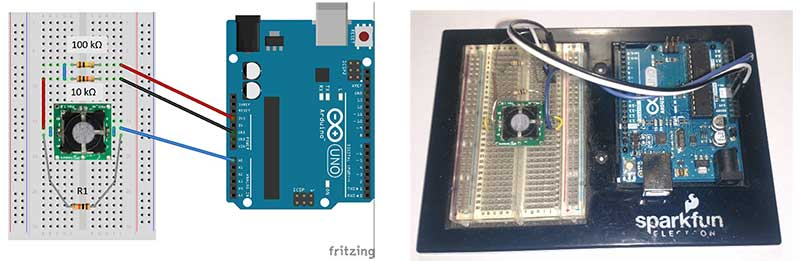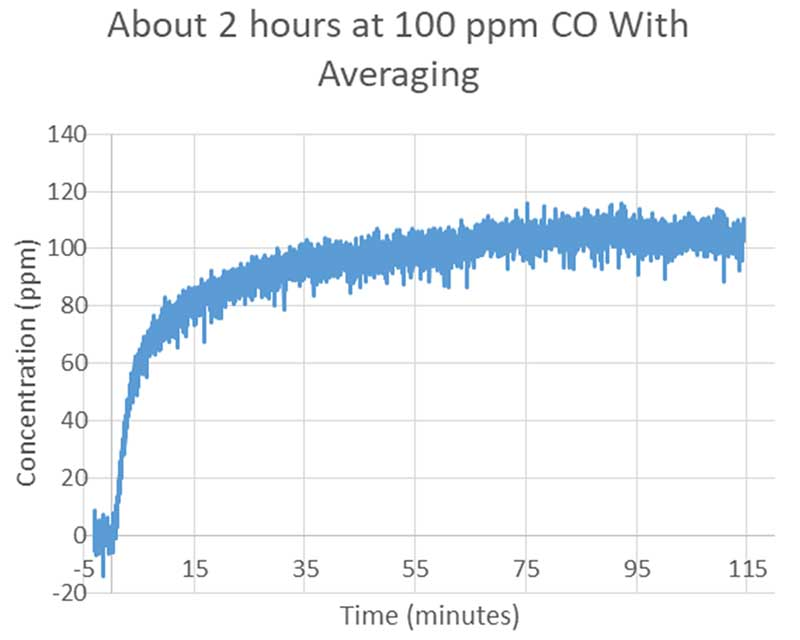The best tools to make your project dreams come true

# Create Your Own CO Detector

## By David PeasleeBuilding a CO (carbon monoxide) detector that can alert you to dangerous levels of CO can be done with as little as a gas sensor, an Arduino (or other microcontroller) and some resistors. Adding a few op-amps can boost the sensitivity to measure the amount of CO present in the environment. Expanding on this knowledge, you can build your own air quality monitoring station, and compare your own backyard with levels reported by local weather stations.

Required Materials

Project Story

While working at a gas sensor company, I worked with many startups that had many great ideas for measuring air quality. While I really hope that everyone will someday have gas sensors in their phones and computers, for now, I'd like to help the everyday Maker build their own air monitoring systems.

For this project, I'd like to start with the basics: What makes an electrochemical sensor work?

• There are two basic types of electrochemical gas sensors, 2-electrode and 3-electrode. They both operate with the same basic principles. A gas diffuses into the sensor and makes its way to the working electrode. There it reacts with a catalyst and creates ions (charged molecules). Those ions move through the electrolyte (sometimes similar to battery acid) and again react at the counter electrode where the electrons are freed (or collected) to move through the circuit. Depending on the gas being reacted and the catalyst, those electrons can be flowing into or out of either electrode. Measuring this flow of electrons (current) we get an idea of how many particles are reacting per second, which gives us an idea of how many particles are there in the first place.
• With the addition of a third electrode, the reference, we can create a constant voltage differential between the reference and working voltages. This is what we call the bias potential. For example, if the reference voltage is 1.25 V, and the working is at 1.35 V we say that the bias is +100 mV. The chemistry has nothing to do with the 1.25 and 1.35 Volts, it only cares that there is a +100 mV potential on the working electrode. There's a lot more that goes into this electrochemical cell, but this is all we really need to know to get started. In this project, we are going to take a 3-electrode sensor and use it in a 2-electrode configuration as in Figure 1.
• One last bit of info, PPM, or parts per million, is a standard of stating the concentration. For example, 1 ppm is one particle of a particular gas per million total particles of gas present.Figure 1. A 3-electrode gas sensor connected in a 2-electrode configuration. (Image courtesy of SPEC Sensors)

Disclaimer 1: Caution: I'm going to show you some results from testing I did with pressurized Carbon Monoxide gas. A small cylinder of CO is available online, but it is lethal. You should read up on this before you decide to use CO in a project.1 Disclaimer 2: If you need to find an alternative source of CO, a cigarette will produce around 300 ppm CO in the inhaled stream.

How-toFigure 2. Basic gas sensor prototype setup. (Image courtesy of SPEC Sensors)

Part 1 - Basic sensor setup

I'm going to start with the most basic circuit and show you how to measure the sensor with just a sensor, a 10 kΩ resistor, and a multimeter.

i. Cut the two pins that are not labeled (see Figure 2), then center the sensor on the breadboard.

ii. Use a short jumper to short the R and C pins (counter and reference). Use another short jumper to short the W1 and W2 pins (this is just giving an extra route for the current as the pins are already shorted on the PCB).

iii. Connect the positive lead of the multimeter to the R/C side of the sensor. Connect the negative side of the meter to the W1/W2 side of the sensor.

iv. Finally use a 10 kΩ resistor (R1) to connect the R/C pins to the W1/W2 pins.

At this point you are ready to test the sensor. You can use a match or smoke, but it will work better if it is in a sealed container. A Pyrex/glass dish with an airtight top will work great. Try lighting a match and then blowing it out and putting it in the dish with the sensor. You may have to cut holes to run the electric lines through. In my setup, I ran 200 ppm CO at about 100 cubic centimeters per minute, which is rather slow. As shown in Figure 3, only the multimeter is hooked up to the sensor.Figure 3. CO sensor setup with only a multimeter connected to show output. (Image courtesy of SPEC Sensors)

Some needed math:

The measurement you can expect is dependent on your setup and the sensor’s sensitivity. In this 2 electrode mode you can expect about half the sensitivity, so if your sensor says 4.0 nA/ppm, the you can expect about 2 nA/ppm. To convert mV to ppm use Ohm’s law: Voltage (V) = Current (A) x Resistance (Ω). For example:

In the Figure 3 setup, I start with 0.2 mV with no gas and end with 4.8 mV with about 200 ppm flowing into the box and out through a vent to outside. The voltage difference is 4.6 mV. The resistor R1 is 10 kΩ so the current is 0.0046 V/ 10,000 Ω, or 460 nA. To get ppm, divide measured current by the sensitivity; 460 nA / ~2 nA/ppm, or 230 ppm. This is pretty close to what I put in the box; 200 ppm. To calculate the new sensitivity, use mV and ppm. My new sensitivity is 4.6 mV/200 ppm or 0.23 mV/ppm.

Figure 4 shows the direction the current travels through the circuit. Terminal A is the positive lead of the DMM and B is the negative (common) lead. You can imagine that electrons are used up at the working electrode and produced at the counter/reference electrode. If the leads of the sensor are in backwards, you will measure a negative voltage instead.Figure 4. Direction of current flow in the sensor setup. (Image courtesy of SPEC Sensors)

Part 2 – Advanced sensor setup

Now we are going to incorporate the Arduino Uno into this project. There are a few changes that we will make to overcome its limitations, but in reality, you can hook it up the same way you did with the multimeter. The positive side will connect analog pin A0 to the R/C pins of the sensor, and the negative side will connect the GND to the W1/W2 pins of the sensor. With this, use analogRead() to measure the voltage across the sensor and resistor R1. The final Arduino code can be found in the at the end of this article, and it includes the sampling improvements that I’ve outlined below.

Notes on the ADC of an Arduino:

From the above calculation, you can see that 1 ppm will generate a voltage of 0.23 mV. That is a problem for the 10-bit ADC of the Arduino, as the minimum resolution for the Uno is 4.88 mV (5 V/1024 levels). That means the minimum the Arduino can detect is 21 ppm, which is still too high.

The first improvement is to change the analog reference to the internal reference of 1.1 V [analogReference(INTERNAL)]. This changes the minimum resolution to 4.7 ppm, which is better. Please read the instructions for this at https://www.arduino.cc/en/Reference/AnalogReference. The next fix is to implement averaging. The maximum reading rate of analogRead() is 10,000 times a second. If you average a bunch of these readings over a second, say 256, that will improve the resolution significantly.2Finally, as you have increased the resolution, you may find that zero is no longer 0 V on the ADC of the Arduino. To fix this, we need to bring that zero voltage (meaning zero current) to a higher value that can be read by the ADC. Here we will implement a voltage ladder with the addition of a 100 kΩ and a 10 kΩ resistor. When there is zero current generated by the sensor, we will be measuring the voltage between the two new resistors. The current through the resistors is 3.3 V/ (100,000 +10,000 Ω), or 30 microAmps. So, between the two new resistors we will be measuring 10,000 Ω * 30 microAmps or 0.3 Volts. This voltage ladder will use 30 microAmps, so if you need to save power, for battery life, try increasing the value of the 100 kΩ resistor. Now our new zero Voltage is near 0.3 Volts, and anything above this is due to CO gas generating a voltage across the resistor R1.Figure 5. CO sensor prototype with an Arduino board. (Image courtesy of SPEC Sensors)

So as a final example, with this setup we are measuring 0.355 Volts. First subtract the zero voltage of 0.3 V, giving 0.055 V or 55 mV. Now divide by our new sensitivity of 0.23 mV/ppm and we are measuring 240 ppm CO, which is a dangerous level to breath for long periods of time.

Summary and next steps:

By using the serial terminal, I was able to capture about 2 hours of logging at about 1 sample every 1.5 seconds. Figure 6 shows what is possible with the Arduino, some resistors, the sensor and some averaging. The next steps would be to use the sensor in a 3-electrode configuration, which provides added stability, and signal amplification. The parts for that include a dual op amp, or two dual op amps, and some resistors. In reality, the sensors output can depend on other factors such as temperature and changes in humidity, but a good weather station will be recording these things as well.Figure 6. Output of the Arduino sensor setup with data averaging. (Image courtesy of SPEC Sensors)

In future articles, I will be presenting other projects, including the 3-electrode circuit and using digital versions of these sensors. If you are ready to build your own circuit you can check out the application notes at SPEC Sensors.

Arduino Code used for this project:

Copy Code
`/*  Analog input, Serial output  Reads an analog gas sensor at pins A0,  Also prints the results to the serial monitor.  The circuit:  Gas sensor pin W (Working) connected to middle of resistor ladder (I used 10k and 100k).  Gas sensor C (counter) and R (reference) connected together and then jumped to analog pin A0.  10 kOhm resistor between W1/W2 and C/R.  created 01 Jul. 2017  by David Peaslee*/// these constants won't change.  They're used to give names to the pins used:const int analogInPin = A0;  // Analog input pin that the sensor is attached toconst int resValue = 9700;  // Value of 10kOhm resistor !change this to match your resistorconst float Vref = 1.1;  //This is the voltage of the internal referenceconst long int cOff = 68286; //286mV offset due to resistor ladder. Try taking the average of a long//measurement of the counts without a sensor in place. This should give a good Zero.const float Sf = 2.11; // sensitivity in nA/ppm,//this is roughly about 1/2 the sensitivity of the barcode on the sensor. Try finding a known//concentration of CO to measure, or just approximate.const int extraBit = 256; //Number of samples averaged, like adding 8 bits to ADClong int sensorValue = 0;        // value read from the sensorfloat currentValue = 0;        // current read from the sensorvoid setup() {  // initialize serial communications at 9600 bps:  Serial.begin(9600);  // !!!set analog reference to 1.1 Volts!!!  analogReference(INTERNAL);}void loop() {  // read the analog in value:  sensorValue = 0;  for (int i = 0; i < extraBit; i++) {    sensorValue = analogRead(analogInPin) + sensorValue;    delay(3);   // needs 2 ms for the analog-to-digital converter to settle after the last reading  }  sensorValue = sensorValue - cOff; //subtract the offset of the resistor ladder * 256.  // print the results to the serial monitor:  Serial.print("PPM = ");  Serial.print( ((float) sensorValue / extraBit / 1024 * Vref / resValue * 1000000000) / Sf);  Serial.print("\tnA = ");  Serial.print( (float) (sensorValue) / extraBit / 1024 * Vref / resValue * 1000000000);  Serial.print("\tCounts = " );  Serial.println(sensorValue);    //Trying to make each loop 1 second  delay(218);  //1 second – 3ms*256ms (each adc read)-14ms (for printing)= 218ms}`

For additional Spec Sensor Libraries to work with various ULP Sensors, go to this link at GitHub: https://github.com/SPEC-Sensors/ULPSM

References: